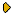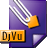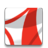Журнал Экспериментальной и Теоретической Физики
 НАЧАЛО | ПОИСК | ДЛЯ АВТОРОВ | ПОМОЩЬОбщая информация о журналеЗолотые страницыАдреса редакцииСодержание журналаСообщения редакцииПравила для авторовЗагрузить статьюПроверить статус статьиЖЭТФ, Том 147, Вып. 1, стр. 174 (Январь 2015)
(Английский перевод - JETP, Vol. 120, No 1, p. 155, January 2015 доступен on-line на www.springer.com )

EXACT SOLUTIONS FOR THE EVOLUTION OF A BUBBLE IN AN IDEAL LIQUID IN A UNIFORM EXTERNAL ELECTRIC FIELD

Поступила в редакцию: 23 Мая 2014

DOI: 10.7868/S0044451015010162DJVU (80.8K)PDF (219.3K)

The dynamics of a bubble in a dielectric liquid under the influence of a uniform external electric field are considered. It is shown that in the situation where the boundary motion is determined only by electrostatic forces, the special regime of fluid motion can be realized for which the velocity and electric field potentials are linearly related. In the two-dimensional case, the corresponding equations are reduced to an equation similar in structure to the well-known Laplacian growth equation, which, in turn, can be reduced to a finite number of ordinary differential equations. This allows us to obtain exact solutions for asymmetric bubble deformations resulting in the formation of a finite-time singularity (cusp).

Сообщить о технических проблемах